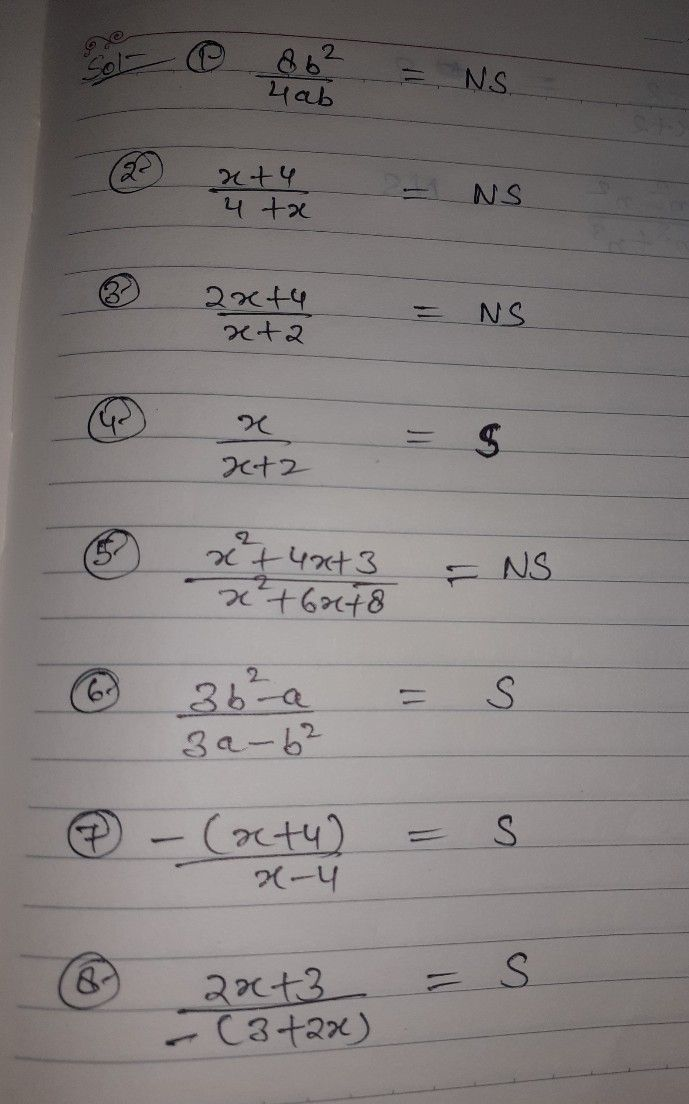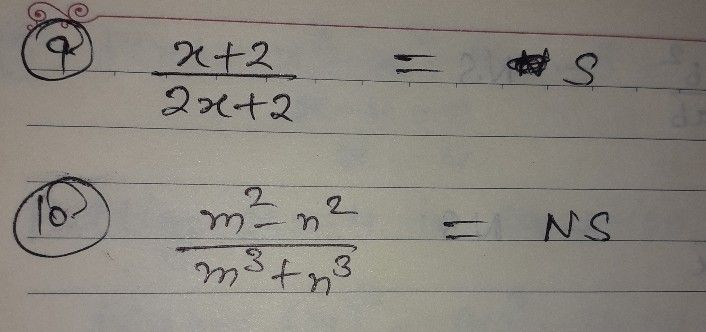Symbol
ProblemActivity $1:$ Simplest, or Not Simplest, that is the Question Identify if the given rational expression is in simplest form or not. Write S if the given is in simplest form otherwise write NS. Write your answer on a separate sheet of paper. 1. $\dfrac {8b^{1}} {4ab}$ $6$ $\dfrac {3b^{2}-a} {3a-b^{2}}$ $2$ $\dfrac {x+4} {4+x}$ $7$ 7. $\dfrac {-\left(x+4\right)} {x-4}$ $3$ $\dfrac {2x+4} {x+2}$ $8$ $\dfrac {2x+3} {-\left(3+2x\right)}$ $4.\dfrac {x} {x+2}$ 9. $\dfrac {x+2} {2x+2}$ $5$ $\dfrac {x^{2}+4x+3} {x^{2}+6x+8}$ $10$ $\dfrac {m^{2}-n^{2}} {m^{3}+n^{3}}$
7th-9th grade
Algebra
Search count: 110
Question content
PLEASE ANSWER THIS ALL READ THE DIRECTION THANKYOU
SolutionQanda teacher - SHIVKUMARCokk
I hope you understand
If you have any problem with answer plz ask me or inform me first.
But If you're satisfied plz do like button and give 5 stars rating as a gift of Thank you...??Student
OKAY THANKYOU SO MUCH?Qanda teacher - SHIVKUMARC
plz evaluate this question